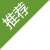## 城市站群

• ### 地区站群# 新闻或产品评论功能实现代码

if(\$cfg_comment == 'Y')
{
?>

\$dosql->Execute("SELECT * FROM `#@__usercomment` WHERE molds=2 AND aid=\$id AND isshow=1 ORDER BY id DESC");
while(\$row = \$dosql->GetArray())
{
echo '
• '.\$row['uname'].'

'.\$row['body'].'

'.GetDateTime(\$row['time']).'
• ';
}
?>

\$r = \$dosql->GetOne("SELECT COUNT(id) as n FROM `#@__usercomment` WHERE molds=2 AND aid=\$id AND isshow=1 ORDER BY id DESC");
echo \$r['n'];
?>

}
}
?>